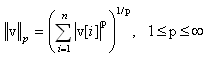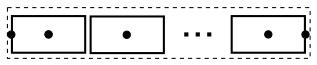# Package Modelica.​Math.​VectorsLibrary of functions operating on vectors

### Information

#### Library content

This library provides functions operating on vectors:

• toString(v) - returns the string representation of vector v.
• isEqual(v1, v2) - returns true if vectors v1 and v2 have the same size and the same elements.
• norm(v,p) - returns the p-norm of vector v.
• length(v) - returns the length of vector v (= norm(v,2), but inlined and therefore usable in symbolic manipulations)
• normalize(v) - returns vector in direction of v with length = 1 and prevents zero-division for zero vector.
• reverse(v) - reverses the vector elements of v.
• sort(v) - sorts the elements of vector v in ascending or descending order.
• find(e, v) - returns the index of the first occurrence of scalar e in vector v.
• interpolate(x, y, xi) - returns the interpolated value in (x,y) that corresponds to xi.
• relNodePositions(nNodes) - returns a vector of relative node positions (0..1).

Matrices

Extends from `Modelica.​Icons.​Package` (Icon for standard packages).

### Package Contents

NameDescription
`find`Find element in a vector
`interpolate`Interpolate linearly in a vector
`isEqual`Determine if two Real vectors are numerically identical
`length`Return length of a vector (better as norm(), if further symbolic processing is performed)
`norm`Return the p-norm of a vector
`normalize`Return normalized vector such that length = 1 and prevent zero-division for zero vector
`normalizeWithAssert`Return normalized vector such that length = 1 (trigger an assert for zero vector)
`relNodePositions`Return vector of relative node positions (0..1)
`reverse`Reverse vector elements (e.g., v becomes last element)
`sort`Sort elements of vector in ascending or descending order
`toString`Convert a real vector in to a string representation
`Utilities`Utility functions that should not be directly utilized by the user

## Function Modelica.​Math.​Vectors.​toStringConvert a real vector in to a string representation

### Information

#### Syntax

```Vectors.toString(v);
Vectors.toString(v,name="",significantDigits=6);
```

#### Description

The function call "`Vectors.toString(v)`" returns the string representation of vector v. With the optional arguments "name" and "significantDigits" a name and the number of the digits are defined. The default values of "name" and "significantDigits" are "" and 6 respectively. If name=="" (empty string) then the prefix "<name> =" is left out at the output-string.

#### Example

```  v = {2.12, -4.34, -2.56, -1.67};
toString(v);
// = "
//           2.12
//          -4.34
//          -2.56
//          -1.67"
toString(v,"vv",1);
// = "vv =
//           2
//          -4
//          -3
//          -2"
```

Extends from `Modelica.​Icons.​Function` (Icon for functions).

### Inputs

TypeNameDescription
`Real``v[:]`Real vector
`String``name`Independent variable name used for printing
`Integer``significantDigits`Number of significant digits that are shown

### Outputs

TypeNameDescription
`String``s`

## Function Modelica.​Math.​Vectors.​isEqualDetermine if two Real vectors are numerically identical

### Information

#### Syntax

```Vectors.isEqual(v1, v2);
Vectors.isEqual(v1, v2, eps=0);
```

#### Description

The function call "`Vectors.isEqual(v1, v2)`" returns true, if the two Real vectors v1 and v2 have the same dimensions and the same elements. Otherwise the function returns false. Two elements e1 and e2 of the two vectors are checked on equality by the test "abs(e1-e2) ≤ eps", where "eps" can be provided as third argument of the function. Default is "eps = 0".

#### Example

```  Real v1 = {1, 2, 3};
Real v2 = {1, 2, 3, 4};
Real v3 = {1, 2, 3.0001};
Boolean result;
algorithm
result := Vectors.isEqual(v1,v2);     // = false
result := Vectors.isEqual(v1,v3);     // = false
result := Vectors.isEqual(v1,v1);     // = true
result := Vectors.isEqual(v1,v3,0.1); // = true
```

Extends from `Modelica.​Icons.​Function` (Icon for functions).

### Inputs

TypeNameDescription
`Real``v1[:]`First vector
`Real``v2[:]`Second vector (may have different length as v1)
`Real``eps`Two elements e1 and e2 of the two vectors are identical if abs(e1-e2) <= eps

### Outputs

TypeNameDescription
`Boolean``result`= true, if vectors have the same length and the same elements

## Function Modelica.​Math.​Vectors.​normReturn the p-norm of a vector

### Information

#### Syntax

```Vectors.norm(v);
Vectors.norm(v,p=2);   // 1 ≤ p ≤ ∞
```

#### Description

The function call "`Vectors.norm(v)`" returns the Euclidean norm "`sqrt(v*v)`" of vector v. With the optional second argument "p", any other p-norm can be computed:Besides the Euclidean norm (p=2), also the 1-norm and the infinity-norm are sometimes used:

 1-norm = sum(abs(v)) norm(v,1) 2-norm = sqrt(v*v) norm(v) or norm(v,2) infinity-norm = max(abs(v)) norm(v,Modelica.Constants.inf)

Note, for any vector norm the following inequality holds:

```norm(v1+v2,p) ≤ norm(v1,p) + norm(v2,p)
```

#### Example

```  v = {2, -4, -2, -1};
norm(v,1);    // = 9
norm(v,2);    // = 5
norm(v);      // = 5
norm(v,10.5); // = 4.00052597412635
norm(v,Modelica.Constants.inf);  // = 4
```

Extends from `Modelica.​Icons.​Function` (Icon for functions).

### Inputs

TypeNameDescription
`Real``v[:]`Real vector
`Real``p`Type of p-norm (often used: 1, 2, or Modelica.Constants.inf)

### Outputs

TypeNameDescription
`Real``result`p-norm of vector v

## Function Modelica.​Math.​Vectors.​lengthReturn length of a vector (better as norm(), if further symbolic processing is performed)

### Information

#### Syntax

```Vectors.length(v);
```

#### Description

The function call "`Vectors.length(v)`" returns the Euclidean length "`sqrt(v*v)`" of vector v. The function call is equivalent to Vectors.norm(v). The advantage of length(v) over norm(v)"is that function length(..) is implemented in one statement and therefore the function is usually automatically inlined. Further symbolic processing is therefore possible, which is not the case with function norm(..).

#### Example

```  v = {2, -4, -2, -1};
length(v);  // = 5
```

Extends from `Modelica.​Icons.​Function` (Icon for functions).

### Inputs

TypeNameDescription
`Real``v[:]`Real vector

### Outputs

TypeNameDescription
`Real``result`Length of vector v

## Function Modelica.​Math.​Vectors.​normalizeReturn normalized vector such that length = 1 and prevent zero-division for zero vector

### Information

#### Syntax

```Vectors.normalize(v);
Vectors.normalize(v,eps=100*Modelica.Constants.eps);
```

#### Description

The function call "`Vectors.normalize(v)`" returns the unit vector "`v/length(v)`" of vector v. If length(v) is close to zero (more precisely, if length(v) < eps), v/eps is returned in order to avoid a division by zero. For many applications this is useful, because often the unit vector e = v/length(v) is used to compute a vector x*e, where the scalar x is in the order of length(v), i.e., x*e is small, when length(v) is small and then it is fine to replace e by v to avoid a division by zero.

Since the function has the "Inline" annotation, it is usually inlined and symbolic processing is applied.

#### Example

```  normalize({1,2,3});  // = {0.267, 0.534, 0.802}
normalize({0,0,0});  // = {0,0,0}
```

Extends from `Modelica.​Icons.​Function` (Icon for functions).

### Inputs

TypeNameDescription
`Real``v[:]`Real vector
`Real``eps`if |v| < eps then result = v/eps

### Outputs

TypeNameDescription
`Real``result[size(v, 1)]`Input vector v normalized to length=1

## Function Modelica.​Math.​Vectors.​normalizeWithAssertReturn normalized vector such that length = 1 (trigger an assert for zero vector)

### Information

#### Syntax

```Vectors.normalizeWithAssert(v);
```

#### Description

The function call "`Vectors.normalizeWithAssert(v)`" returns the unit vector "`v/sqrt(v*v)`" of vector v. If vector v is a zero vector, an assert is triggered.

Since the function has the "Inline" annotation, it is usually inlined and symbolic processing is applied.

#### Example

```  normalizeWithAssert({1,2,3});  // = {0.267, 0.534, 0.802}
normalizeWithAssert({0,0,0});  // error (an assert is triggered)
```

Extends from `Modelica.​Icons.​Function` (Icon for functions).

### Inputs

TypeNameDescription
`Real``v[:]`Real vector

### Outputs

TypeNameDescription
`Real``result[size(v, 1)]`Input vector v normalized to length=1

## Function Modelica.​Math.​Vectors.​reverseReverse vector elements (e.g., v becomes last element)

### Information

#### Syntax

```Vectors.reverse(v);
```

#### Description

The function call "`Vectors.reverse(v)`" returns the vector elements in reverse order.

#### Example

```  reverse({1,2,3,4});  // = {4,3,2,1}
```

Extends from `Modelica.​Icons.​Function` (Icon for functions).

### Inputs

TypeNameDescription
`Real``v[:]`Real vector

### Outputs

TypeNameDescription
`Real``result[size(v, 1)]`Elements of vector v in reversed order

## Function Modelica.​Math.​Vectors.​sortSort elements of vector in ascending or descending order

### Information

#### Syntax

```           sorted_v = Vectors.sort(v);
(sorted_v, indices) = Vectors.sort(v, ascending=true);
```

#### Description

Function sort(..) sorts a Real vector v in ascending order and returns the result in sorted_v. If the optional argument "ascending" is false, the vector is sorted in descending order. In the optional second output argument the indices of the sorted vector with respect to the original vector are given, such that sorted_v = v[indices].

#### Example

```  (v2, i2) := Vectors.sort({-1, 8, 3, 6, 2});
-> v2 = {-1, 2, 3, 6, 8}
i2 = {1, 5, 3, 4, 2}
```

Extends from `Modelica.​Icons.​Function` (Icon for functions).

### Inputs

TypeNameDescription
`Real``v[:]`Real vector to be sorted
`Boolean``ascending`= true if ascending order, otherwise descending order

### Outputs

TypeNameDescription
`Real``sorted_v[size(v, 1)]`Sorted vector
`Integer``indices[size(v, 1)]`sorted_v = v[indices]

## Function Modelica.​Math.​Vectors.​findFind element in a vector

### Information

#### Syntax

```Vectors.find(e, v);
Vectors.find(e, v, eps=0);
```

#### Description

The function call "`Vectors.find(e, v)`" returns the index of the first occurrence of input e in vector v. The test of equality is performed by "abs(e-v[i]) ≤ eps", where "eps" can be provided as third argument of the function. Default is "eps = 0".

#### Example

```  Real v = {1, 2, 3};
Real e1 = 2;
Real e2 = 3.01;
Boolean result;
algorithm
result := Vectors.find(e1,v);          // = 2
result := Vectors.find(e2,v);          // = 0
result := Vectors.find(e2,v,eps=0.1);  // = 3
```

Extends from `Modelica.​Icons.​Function` (Icon for functions).

### Inputs

TypeNameDescription
`Real``e`Search for e
`Real``v[:]`Real vector
`Real``eps`Element e is equal to a element v[i] of vector v if abs(e-v[i]) <= eps

### Outputs

TypeNameDescription
`Integer``result`v[result] = e (first occurrence of e); result=0, if not found

## Function Modelica.​Math.​Vectors.​interpolateInterpolate linearly in a vector

### Information

#### Syntax

```// Real    x[:], y[:], xi, yi;
// Integer iLast, iNew;
yi = Vectors.interpolate(x,y,xi);
(yi, iNew) = Vectors.interpolate(x,y,xi,iLast=1);
```

#### Description

The function call "`Vectors.interpolate(x,y,xi)`" interpolates linearly in vectors (x,y) and returns the value yi that corresponds to xi. Vector x[:] must consist of monotonically increasing values. If xi < x or > x[end], then extrapolation takes places through the first or last two x[:] values, respectively. If the x and y vectors have length 1, then always y is returned. The search for the interval x[iNew] ≤ xi < x[iNew+1] starts at the optional input argument "iLast". The index "iNew" is returned as output argument. The usage of "iLast" and "iNew" is useful to increase the efficiency of the call, if many interpolations take place. If x has two or more identical values then interpolation utilizes the x-value with the largest index.

#### Example

```  Real x1[:] = { 0,  2,  4,  6,  8, 10};
Real x2[:] = { 1,  2,  3,  3,  4,  5};
Real y[:]  = {10, 20, 30, 40, 50, 60};
algorithm
(yi, iNew) := Vectors.interpolate(x1,y,5);  // yi = 35, iNew=3
(yi, iNew) := Vectors.interpolate(x2,y,4);  // yi = 50, iNew=5
(yi, iNew) := Vectors.interpolate(x2,y,3);  // yi = 40, iNew=4
```

Extends from `Modelica.​Icons.​Function` (Icon for functions).

### Inputs

TypeNameDescription
`Real``x[:]`Abscissa table vector (strict monotonically increasing values required)
`Real``y[size(x, 1)]`Ordinate table vector
`Real``xi`Desired abscissa value
`Integer``iLast`Index used in last search

### Outputs

TypeNameDescription
`Real``yi`Ordinate value corresponding to xi
`Integer``iNew`xi is in the interval x[iNew] <= xi < x[iNew+1]

## Function Modelica.​Math.​Vectors.​relNodePositionsReturn vector of relative node positions (0..1)

### Information

#### Syntax

```Vectors.relNodePositions(nNodes);
```

#### Description

The function call "`relNodePositions(nNodes)`" returns a vector with the relative positions of the nodes of a discretized pipe with nNodes nodes (including the node at the left and at the right side of the pipe), see next figure:#### Example

```  Real xsi;
algorithm
xsi = relNodePositions(7);  // xsi = {0, 0.1, 0.3, 0.5, 0.7, 0.9, 1}
```

Extends from `Modelica.​Icons.​Function` (Icon for functions).

### Inputs

TypeNameDescription
`Integer``nNodes`Number of nodes (including node at left and right position)

### Outputs

TypeNameDescription
`Real``xsi[nNodes]`Relative node positions

Generated 2018-12-12 12:14:34 EST by MapleSim.